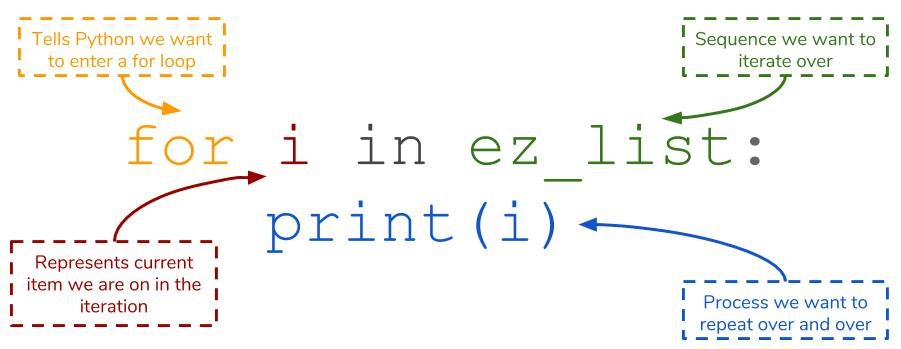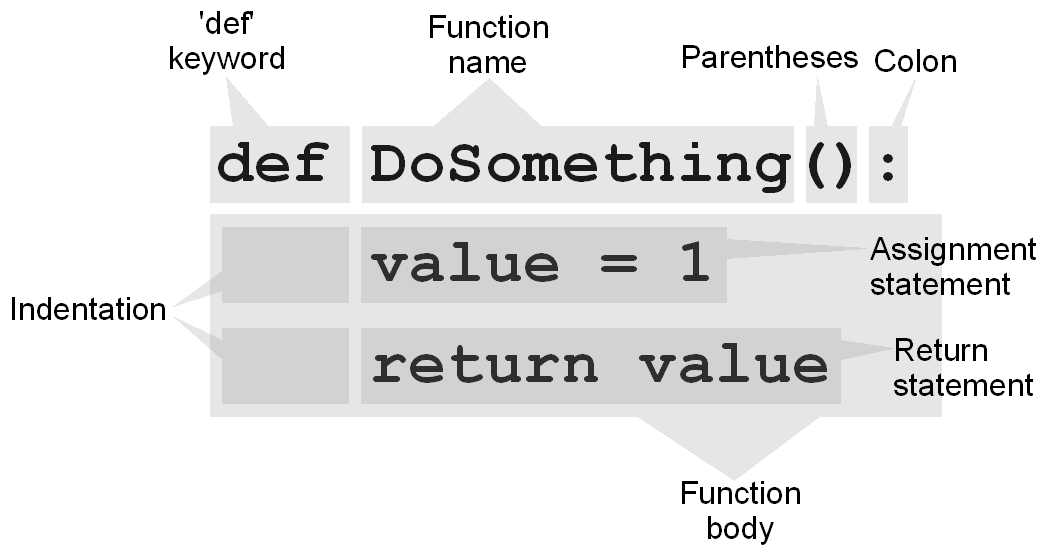Learn To Code In Python

Teaches you how to code in python. By `PYer`
This tutorial excpects some basic knowledge of coding in another language.

# What is python?

Python is a very popular coding language. Little people use it for serious projects, but it is still useful to learn. It was created in 1991 by `Guido van Rossum`.
Look at a few uses of python:

• Desktop Applications
• Web Applications
• Complex Scientific Equations

Let's look at a few reasons why it is useful:

• Compatible with other systems/platforms
• Millions of useful modules

These are just a few, you can find a bunch more by researching it.

# Know This Before We Start

What we will be teaching you is specifically `python 3`. This is the most updated version, but the version 2 is still widely used.
Here we will be using replit, but there are multiple text editors you can find.

# Python Syntax

Python syntax was made for readability, and easy editing. For example, the python language uses a `:` and indented code, while javascript and others generally use `{}` and indented code.

# First Program

Lets create a python 3 repl, and call it Hello World. Now you have a blank file called main.py. Now let us write our first line of code:

helloworld.py

``print('Hello world!')``

Brian Kernighan actually wrote the first "Hello, World!" program as part of the documentation for the BCPL programming language developed by Martin Richards.

Now, press the run button, which obviously runs the code. If you are not using replit, this will not work. You should research how to run a file with your text editor.

# Command Line

If you look to your left at the console where hello world was just printed, you can see a `>`, `>>>`, or `\$` depending on what you are using. After the prompt, try typing a line of code.

``````Python 3.6.1 (default, Jun 21 2017, 18:48:35)
[GCC 4.9.2] on linux
> print('Testing command line')
Testing command line
> print('Are you sure this works?')
Are you sure this works?
> ``````

The command line allows you to execute single lines of code at a time. It is often used when trying out a new function or method in the language.

Another cool thing that you can generally do with all languages, are comments. In python, a comment starts with a `#`. The computer ignores all text starting after the `#`.

shortcom.py

``# Write some comments!``

If you have a huge comment, do not comment all the 350 lines, just put `'''` before it, and `'''` at the end. Technically, this is not a comment but a string, but the computer still ignores it, so we will use it.

longcom.py

``````'''
Dear PYer,
I am confused about how you said you could use triple quotes to make
SUPER
LONG
!

I am wondering if this is true,
and if so,
I am wondering if this is correct.

Could you help me with this?

Thanks,
Random guy who used your tutorial.
'''
print('Testing')``````

# New: Variables!

Unlike many other languages, there is no `var`, `let`, or `const` to declare a variable in python. You simply go `name = 'value'`.

vars1.py

``````x = 5
y = 7
z = x*y # 35
print(z) # => 35``````

Remember, there is a difference between integers and strings. Remember: String = `""`. To convert between these two, you can put an int in a `str()` function, and a string in a `int()` function. There is also a less used one, called a float. Mainly, these are integers with decimals. Change them using the `float()` command.

vars2.py

``````x = 5
x = str(x)
b = '5'
b = int(b)
print('x = ', x, '; b = ', str(b), ';') # => x = 5; b = 5;``````

Instead of using the `,` in the print function, you can put a `+` to combine the variables and string.

# Operators

There are many operators in python:

• `+`
• `-`
• `/`
• `*`
These operators are the same in most languages, and allow for addition, subtraction, division, and multiplicaiton.
Now, we can look at a few more complicated ones:
• `%`
• `//`
• `**`
• `+=`
• `-=`
• `/=`
• `*=`
Research these if you want to find out more...

simpleops.py

``````x = 4
a = x + 1
a = x - 1
a = x * 2
a = x / 2``````

You should already know everything shown above, as it is similar to other languages. If you continue down, you will see more complicated ones.

complexop.py

``````a += 1
a -= 1
a *= 2
a /= 2``````

The ones above are to edit the current value of the variable.
Sorry to JS users, as there is no `i++;` or anything.

Fun Fact:
The python language was named after Monty Python.

If you really want to know about the others, view Py Operators

# More Things With Strings

Like the title?
Anyways, a `'` and a `"` both indicate a string, but do not combine them!

quotes.py

``````x = 'hello' # Good
x = "hello" # Good
x = "hello' # ERRORRR!!!``````

slicing.py

### String Slicing

You can look at only certain parts of the string by slicing it, using `[num:num]`.
The first number stands for how far in you go from the front, and the second stands for how far in you go from the back.

``````x = 'Hello everybody!'
x # 'H'
x[-1] # '!'
x # ' '
x[1:] # 'ello everybody!'
x[:-1] # 'Hello everybod'
x[2:-3] # 'llo everyb'``````

### Methods and Functions

Here is a list of functions/methods we will go over:

• `.strip()`
• `len()`
• `.lower()`
• `.upper()`
• `.replace()`
• `.split()`

I will make you try these out yourself. See if you can figure out how they work.

strings.py

``````x = " Testing, testing, testing, testing       "
print(x.strip())
print(len(x))
print(x.lower())
print(x.upper())
print(x.replace('test', 'runn'))
print(x.split(','))``````

Good luck, see you when you come back!

# New: Input()

Input is a function that gathers input entered from the user in the command line. It takes one optional parameter, which is the users prompt.

inp.py

``````print('Type something: ')
x = input()
print('Here is what you said: ', x)``````

If you wanted to make it smaller, and look neater to the user, you could do...

inp2.py

``print('Here is what you said: ', input('Type something: '))``

Running:
inp.py

``````Type something:
Hello World
Here is what you said: Hello World``````

inp2.py

``````Type something: Hello World
Here is what you said: Hello World``````

# New: Importing Modules

Python has created a lot of functions that are located in other .py files. You need to import these modules to gain access to the,, You may wonder why python did this. The purpose of separate modules is to make python faster. Instead of storing millions and millions of functions, , it only needs a few basic ones. To import a module, you must write `input <modulename>`. Do not add the .py extension to the file name. In this example , we will be using a python created module named random.

module.py

``import random``

Now, I have access to all functions in the random.py file. To access a specific function in the module, you would do `<module>.<function>`. For example:

module2.py

``````import random
print(random.randint(3,5)) # Prints a random number between 3 and 5``````

Pro Tip:
Do `from random import randint` to not have to do `random.randint()`, just `randint()`
To import all functions from a module, you could do `from random import *`

# New: Loops!

Loops allow you to repeat code over and over again. This is useful if you want to print Hi with a delay of one second 100 times.

#### `for` Loop

The for loop goes through a list of variables, making a seperate variable equal one of the list every time.
Let's say we wanted to create the example above.

loop.py

``````from time import sleep
for i in range(100):
print('Hello')
sleep(.3)``````

This will print Hello with a .3 second delay 100 times. This is just one way to use it, but it is usually used like this:

loop2.py

``````import time
for number in range(100):
print(number)
time.sleep(.1)``````#### `while` Loop

The while loop runs the code while something stays true. You would put `while <expression>`. Every time the loop runs, it evaluates if the expression is True. It it is, it runs the code, if not it continues outside of the loop. For example:

while.py

``````while True: # Runs forever
print('Hello World!')``````

Or you could do:

while2.py

``````import random
position = '<placeholder>'
while position != 1: # will run at least once
position = random.randint(1, 10)
print(position)``````

# New: `if` Statement

The if statement allows you to check if something is True. If so, it runs the code, if not, it continues on. It is kind of like a while loop, but it executes only once. An if statement is written:

if.py

``````import random
num = random.randint(1, 10)
if num == 3:
print('num is 3. Hooray!!!')
if num > 5:
print('Num is greater than 5')
if num == 12:
print('Num is 12, which means that there is a problem with the python language, see if you can figure it out. Extra credit if you can figure it out!')``````

Now, you may think that it would be better if you could make it print only one message. Not as many that are True. You can do that with an `elif` statement:

elif.py

``````import random
num = random.randint(1, 10)
if num == 3:
print('Num is three, this is the only msg you will see.')
elif num > 2:
print('Num is not three, but is greater than 1')``````

Now, you may wonder how to run code if none work. Well, there is a simple statement called `else:`

else.py

``````import random
num = random.randint(1, 10)
if num == 3:
print('Num is three, this is the only msg you will see.')
elif num > 2:
print('Num is not three, but is greater than 1')
else:
print('No category')``````

# New: Functions (`def`)

So far, you have only seen how to use functions other people have made. Let use the example that you want to print the a random number between 1 and 9, and print different text every time.
It is quite tiring to type:

Characters: 389

nofunc.py

``````import random
print(random.randint(1, 9))
print('Wow that was interesting.')
print(random.randint(1, 9))
print('Look at the number above ^')
print(random.randint(1, 9))
print('All of these have been interesting numbers.')
print(random.randint(1, 9))
print("these random.randint's are getting annoying to type")
print(random.randint(1, 9))
print('Hi')
print(random.randint(1, 9))
print('j')``````

Now with functions, you can seriously lower the amount of characters:

Characters: 254

functions.py

``````import random
def r(t):
print(random.randint(1, 9))
print(t)
r('Wow that was interesting.')
r('Look at the number above ^')
r('All of these have been interesting numbers.')
r("these random.randint's are getting annoying to type")
r('Hi')
r('j')``````There you go! Try making your own functions!

# The End

Now you know all of the basics of python. Congratulations!